Question

# If you move away from a stationary source of a sound, will the frequency be higher...

If you move away from a stationary source of a sound, will the frequency be higher than if you heard the sound while at rest?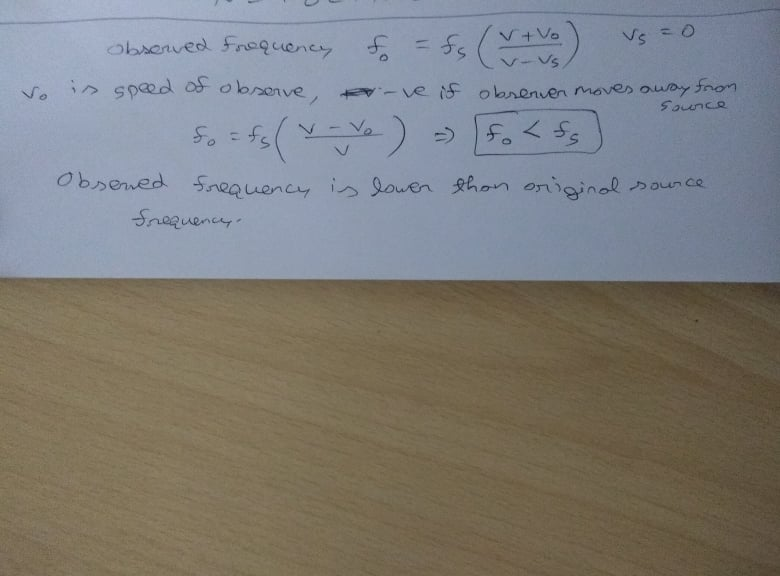#### Earn Coins

Coins can be redeemed for fabulous gifts.

Similar Homework Help Questions
• ### Doppler Effect: Riding a Bicycle Away from a Stationary Source of Sound

You are riding your bicycle directly away from a stationary source of sound and hear a frequency that is 1.4% lower than the emitted frequency. If the speed of soundis 344 m/s, what is your speed?

• ### When the source of a wave and an observer move either towards or away from each...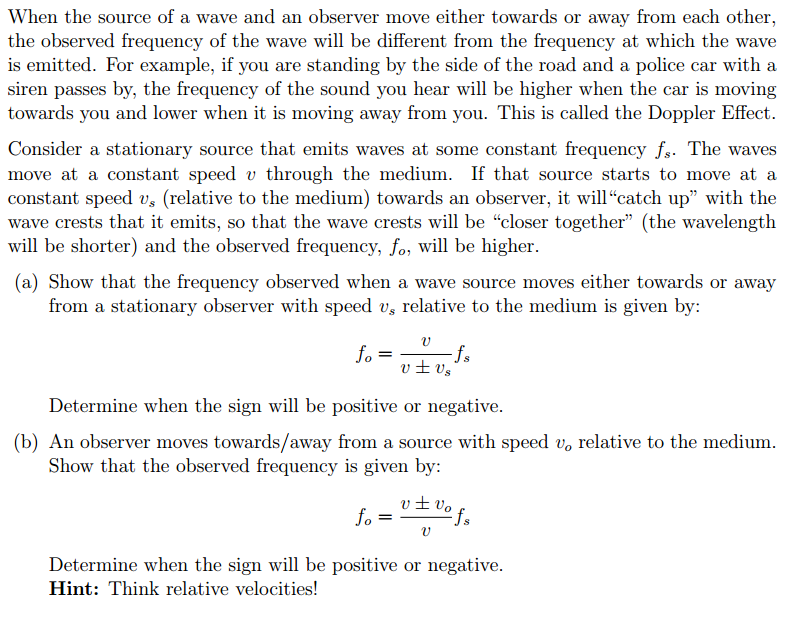When the source of a wave and an observer move either towards or away from each other the observed frequency of the wave will be different from the frequency at which the wave is emitted. For example, if you are standing by the side of the road and a police car with a siren passes by, the frequency of the sound you hear will be higher when the car is moving towards you and lower when it is moving away...

• ### Tutorial Sound Waves . A sound source which produces sound of frequency 300 Hz moves along...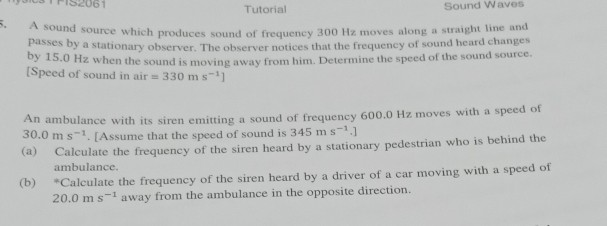Tutorial Sound Waves . A sound source which produces sound of frequency 300 Hz moves along a straight line and passes by a stationary observer. The observer notices that the frequency of sound heard change Speed of sound in air 330 m s-1] 0when the sound is moving away from him. Determine the speed of the sound source. its siren emitting a sound of frequency 600.0 Hz moves with a speed of me that the speed of sound is 345...

• ### 019: A source emits sound with a frequency of 1000 Hz. An observer is moving at...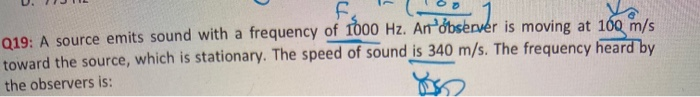019: A source emits sound with a frequency of 1000 Hz. An observer is moving at 160 m/s toward the source, which is stationary. The speed of sound is 340 m/s. The frequency heard by the observers is:

• ### ​A stationary man hears a sound of frequency 447 Hz coming from a source moving towards him

A stationary man hears a sound of frequency 447 Hz coming from a source moving towards him. The frequency of sound from the source is 385 Hz. Calculate the speed of the source in units of m/s. Assume v = 340 m/s.

• ### A source of sound waves of frequency 1.2 kHz is traveling through the air at 0.45 times the speed...

A source of sound waves of frequency 1.2 kHz is traveling through the air at 0.45 times the speed of sound. (a) Find the frequency of the sound received by a stationary observer if the source moves toward her. Hz (b) Repeat if the source moves away from her instead. Hz

• ### Homework 7.2 The sound frequency a listener hear will be shifted to a higher value if...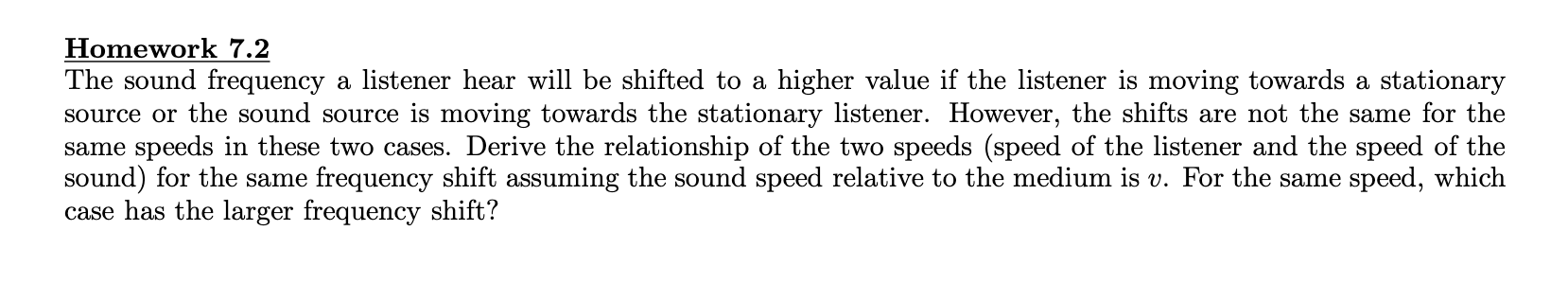Homework 7.2 The sound frequency a listener hear will be shifted to a higher value if the listener is moving towards a stationary source or the sound source is moving towards the stationary listener. However, the shifts are not the same for the same speeds in these two cases. Derive the relationship of the two speeds (speed of the listener and the speed of the sound) for the same frequency shift assuming the sound speed relative to the medium is...

• ### lllllllllllllll esc Problem 3 A sound source is moving toward you such that the frequency you...lllllllllllllll esc Problem 3 A sound source is moving toward you such that the frequency you observe is 5.0% greater than the frequency at the source. Assume that you are at rest. Calculate the speed of the source in km/h. (Take the speed of sound to be 340 m/s.)

• ### 9. The speed of sound was measured in a block of using a sound source and a detector, as shown, The source travels through the block and was detected at the opposite end. From this information, t...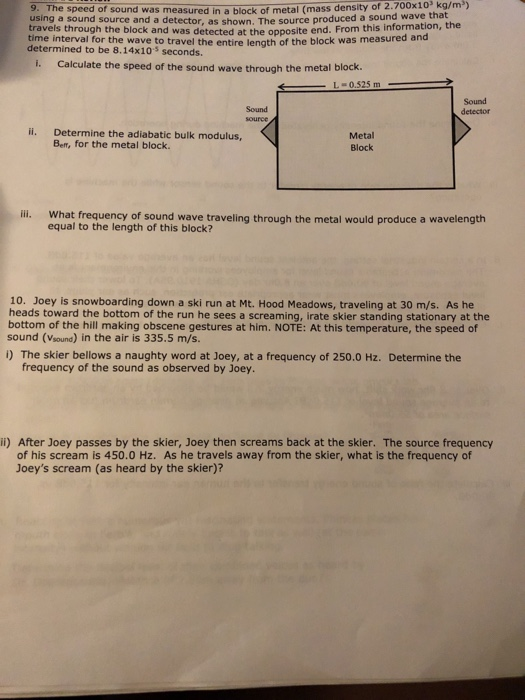9. The speed of sound was measured in a block of using a sound source and a detector, as shown, The source travels through the block and was detected at the opposite end. From this information, the time interval for the wave to travel the entire length of the block was measured and metal (mass density of 2.700x10 kg/m produced a sound wave that determined to be 8.14x10s seconds. i. Calculate the speed of the sound wave through the metal...

• ### The ratio speed of the source to the speed of sound

A stationary detector measures the frequency of a sound source that first moves at constant velocity directly toward the detector and then (after passing the detector)directly away from it. The emitted frequency is f. During the approach the detected frequency is f'app and during the recession it is f'rec. If ( f'app - f'rec)/f =0.750, what is the ratio vs /v of the speed of the source to the speed of sound?